## A visualisation of the effects of conjugation; dienes and biaryls.

Here is another exploration of simple chemical concepts using crystal structures. Consider a simple diene: how does the central C-C bond length respond to the torsion angle between the two C=C bonds?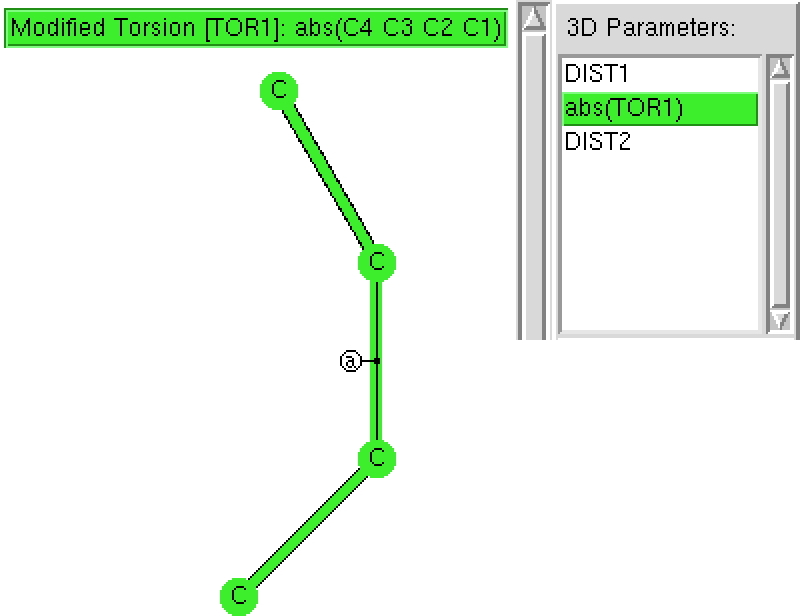The search of the CSD (Cambridge structure database) is constrained to R < 5%, no errors and no disorder and the central  C-C bond is specific to be acyclic.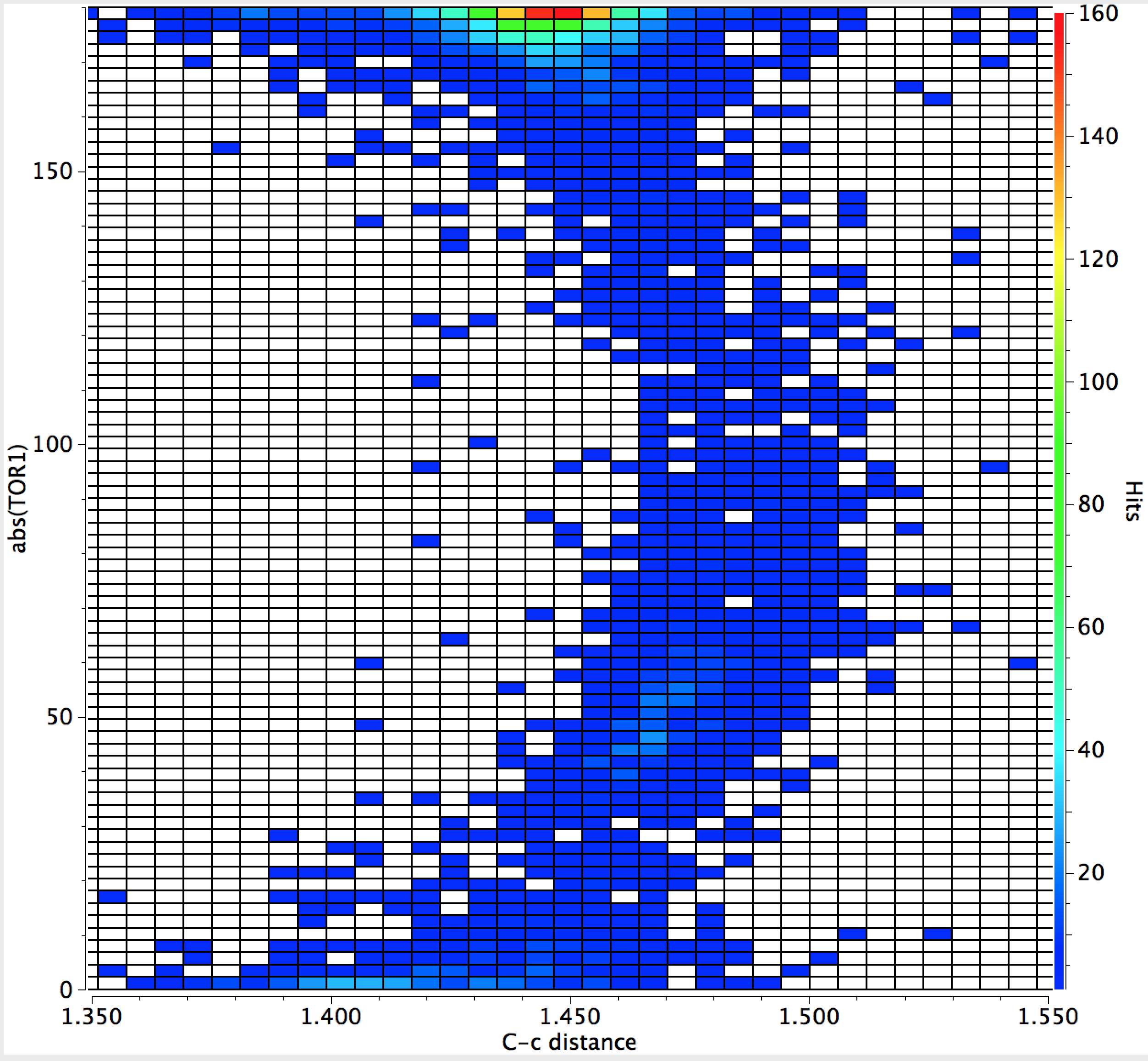1. Note first that the hotspot occurs for a torsion angle of 180°, a trans diene.
2. There is just a hint that the C-C distance for a cis-diene might be a little shorter than the trans diene, but this might not be significant.
3. There is a gentle curve illustrating that the C-C distance is indeed a maximum at 90°
4. The C-C bond extends from ~1.445Å when the two double bonds are coplanar (fully conjugated) to ~1.48Å when orthogonal. Not much of a change, but statistically highly significant.

Here is another search, this time of the C=C-C=C motif embedded into a biaryl, of which there are far more examples. This time, the (red) hotspot is actually at 90°, with local (green) hotspots at 0 and 180° but also at 45 and 135°. Again, you can easily spot the maximum in C-C bond length at 90° but notice how much smaller the bond lengthening is (~ 0.01Å). This lengthening is inhibited by retention of the aromaticity of the two aryl rings; again the statistical effect is highly significant. Perhaps also significant is that the  C-C bond at torsions of 0 or 180° appear to be no shorter than the values at 45 and 135°.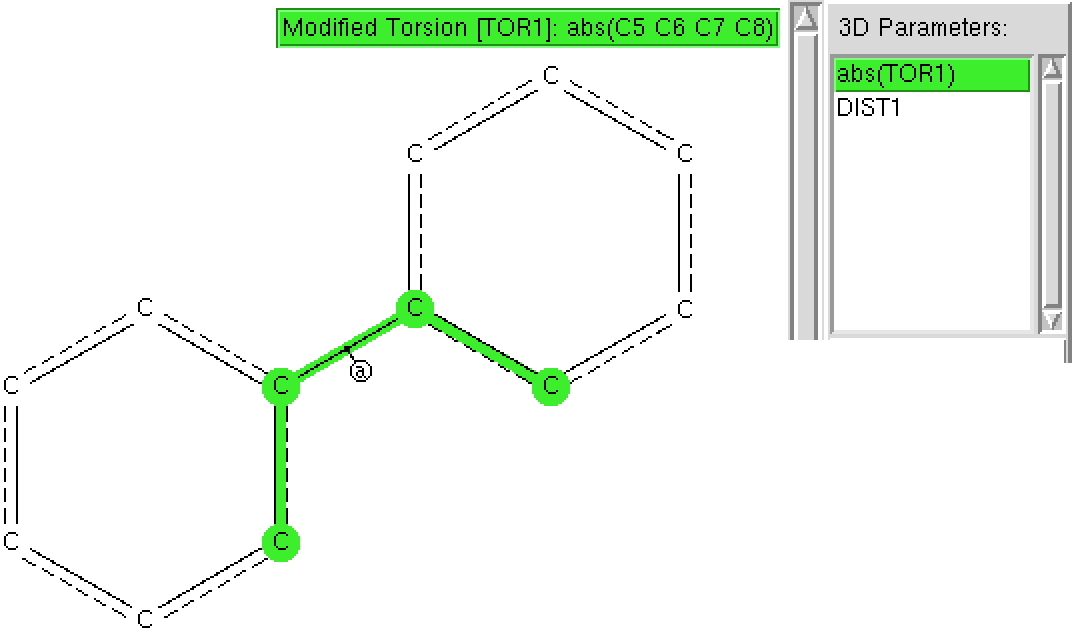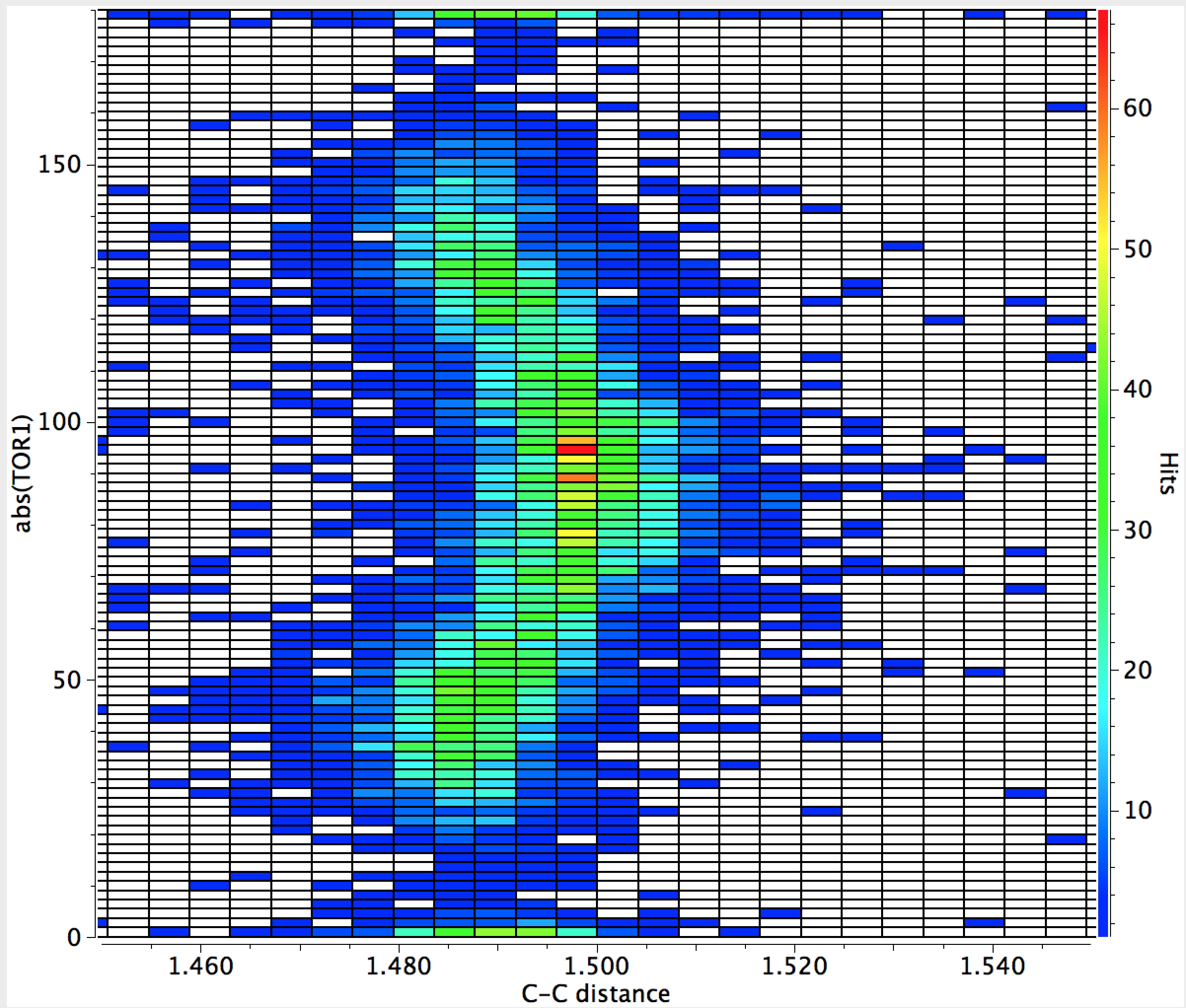Both these searches took about  5 minutes each, and serve to illustrate just how many basic chemical concepts can be teased out of a statistical analysis of crystal structures.

The analogous diagram for O=C-C=C is shown below;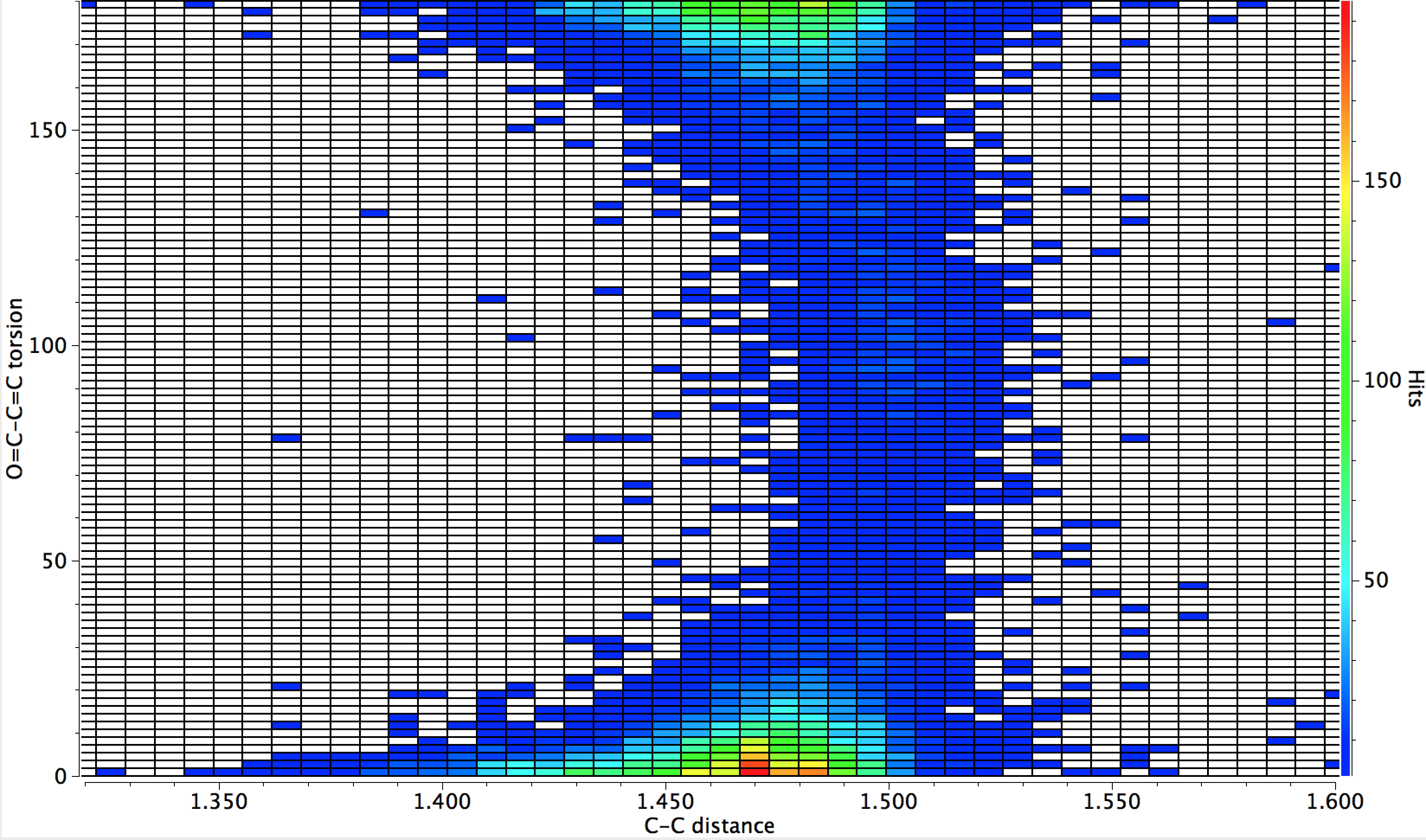That for  O=C-C=O is different however;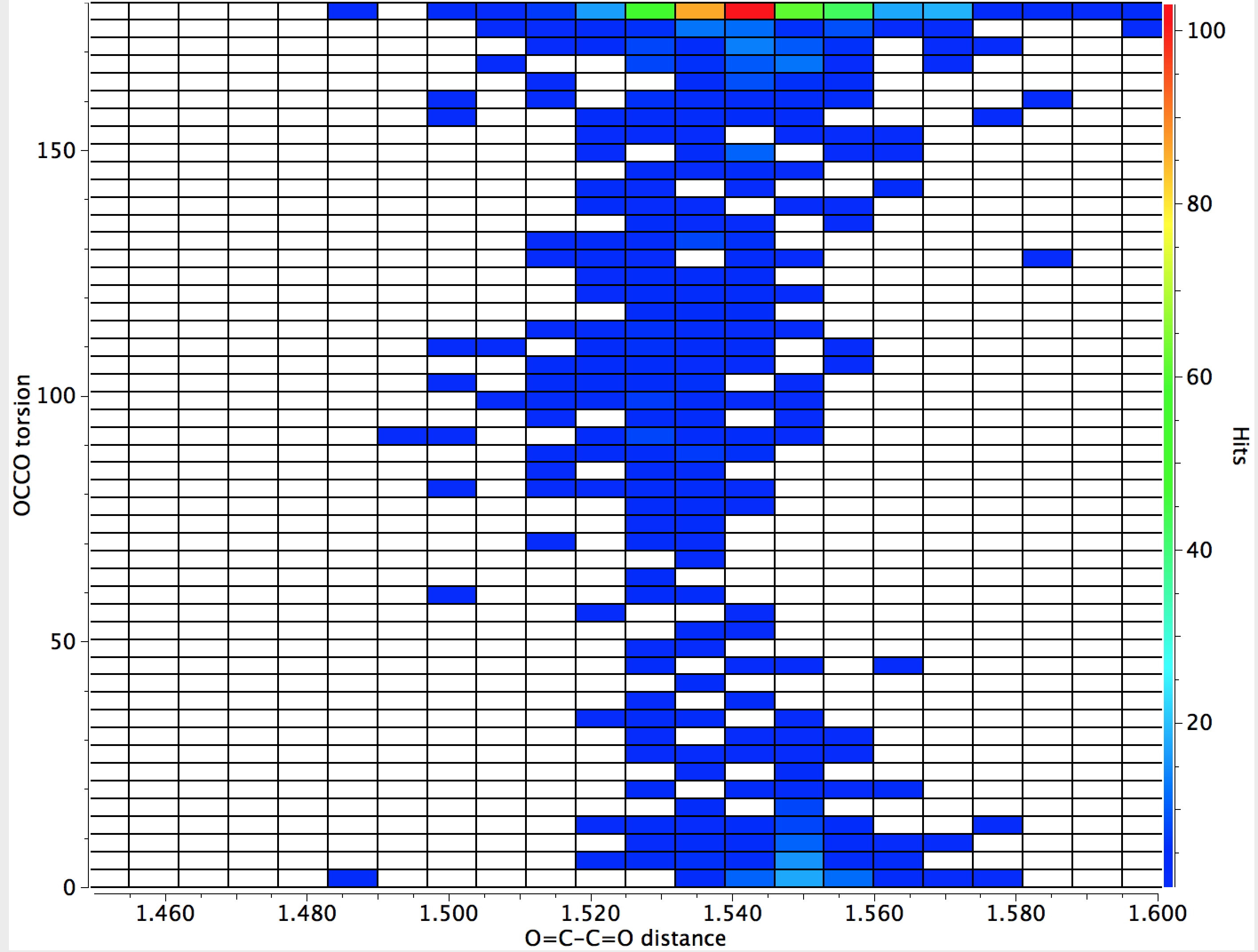#### Acknowledgments

This post has been cross-posted in PDF format at Authorea.